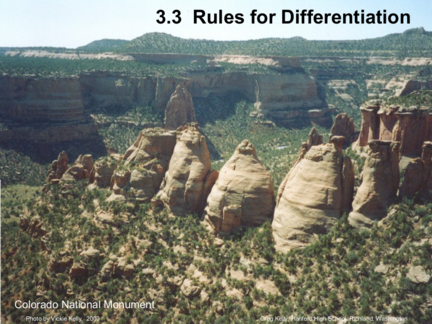# Rules for DifferentiationContributed by:We will discuss some basic rules for differentiation.
1. 3.3 Rules for Differentiation
Photo by Vickie Kelly, 2003 Greg Kelly, Hanford High School, Richland, Washington
2. If the derivative of a function is its slope, then for a
constant function, the derivative must be zero.
d example: y 3
 c  0
dx y 0
The derivative of a constant is zero.

3. If we find derivatives with the difference quotient:
2
d 2
x lim
 x  h  x
lim
2
 x 2
 2 xh  h 2
  x 2
2x
dx h 0 h h 0 h 1
1 1
3
d 3  x  h   x3 1 2 1
x lim 1 3 3 1
dx h 0 h 1 4 6 4 1
2
lim
 x 3
 3 x 2
h  3 xh 2
 h  3
 x 3
3x 2
1 5 10 10 5 1
h 0 h (Pascal’s Triangle)
2 3
d 4
x lim
 x4
 4 x h  3
6 x h  2
4 xh2
 h   x34 4
4x3
dx h 0 h
We observe a pattern: 2x 3x 2 4x 3 5x 4 6x 5 …

4. We observe a pattern: 2x 3x 2 4x 3 5x 4 6x 5 …
examples:
d n
dx
 x  nx n 1
f  x  x 4 y  x8
f  x  4 x 3 y 8 x 7
power rule

5. constant multiple rule:
examples:
d du
 cu  c d n
cx cnx n  1
dx dx
dx
d
7 x5 7 5 x 4 35 x 4
dx
When we used the difference quotient, we observed that
since the limit had no effect on a constant coefficient,
that the constant could be factored to the outside.

6. constant multiple rule:
d du
 cu  c
dx dx
sum and difference rules:
d du dv d du dv
 u  v    u  v  
dx dx dx dx dx dx
4 2
4
y  x  12 x y x  2 x  2
(Each term
dyis treated separately)
3 3
y 4 x  12 4 x  4 x
dx 
7. Find the horizontal tangents of: y x 4  2 x 2  2
dy
4 x 3  4 x
dx
Horizontal tangents occur when slope = zero.
4 x 3  4 x 0 Plugging the x values into the
original equation, we get:
x 3  x 0
y 2, y 1, y 1
x  x  1 0
2
(The function is even, so we
x  x  1  x  1 0 only get two horizontal
tangents.)
x 0,  1, 1

8.
9. y x 4  2 x 2  2
10. y x 4  2 x 2  2
y 2
11. y x 4  2 x 2  2
y 2
y 1
12. y x 4  2 x 2  2
13. y x 4  2 x 2  2
dy
4 x3  4 x
dx
First derivative
(slope) is zero at:
x 0,  1, 1

14. product rule:
d dv du Notice that this is not just the
 uv  u  v
dx dx dx product of two derivatives.
This is sometimes memorized as: d  uv  u dv  v du
d  2 3
dx 
x  3 
2 x  5 x  
  x 2  3  6 x 2  5    2 x 3  5 x   2x 
 
2 x 5  5 x3  6 x3  15 x
 
d 6 x 4  5 x 2  18 x 2  15  4 x 4  10 x 2
dx

2 x 5  11x 3 15 x 
10 x 4  33x 2  15 10 x 4  33 x 2  15

15. quotient rule:
du dv
v u  u  v du  u dv
d u dx dx or d  
   2
dx  v  v2 v
  v
3
d 2 x  5x

    
x 2  3 6 x 2  5  2 x3  5 x  2 x 
2
dx x  3 2
 x  3
2

16. Higher Order Derivatives:
dy
y  is the first derivative of y with respect to x.
dx
dy d dy d 2 y is the second derivative.
y    2
dx dx dx dx (y double prime)
dy 
y  is the third derivative. We will learn
dx later what these
higher order
d derivatives are
 4
y  y is the fourth derivative. used for.
dx